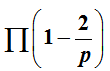#### 期刊菜单

Φ(m)函数的性质
The Nature of the Φ(m) Function
DOI: 10.12677/AAM.2020.91005, PDF, HTML, XML, 下载: 1,952  浏览: 5,260

Abstract: In number theory, for the continuous product formula, the meaning is unclear. This paper gives the definition and nature of Φ(m) function, as well as the relationship between Φ(m) and Euler’s totient function φ(m). As is known to all, Euler function φ(m) is widely used, Φ(m) function if there are other applications, some attempts are made in this paper.

1. 定义与性质

1) 欧拉φ(m)函数

$\phi \left(m\right)=m\prod \left(1-\frac{1}{p}\right)$ ( ${\prod }^{\text{​}}$ 为连乘积符号，p为m的素因子)。

Φ(m)函数   ：

$k={2}^{n}$$\Phi \left(m\right)=\frac{m}{2}\prod \left(1-\frac{2}{p}\right)$ ( ${\prod }^{\text{​}}$ 为连乘积符号，p为m的奇素因子)。

$m={2}^{n}$$\Phi \left(m\right)=\phi \left(m\right)=\frac{m}{2}$

2) 若m为奇数(m ≥ 3)，函数Φ(m)是小于m的正整数中q与m互质且q − k或q + k (k ≥ 1)与m互质的正整数q的数目。

$k={2}^{n}$$\Phi \left(m\right)=m\prod \left(1-\frac{2}{p}\right)$ ( ${\prod }^{\text{​}}$ 为连乘积符号，p为m的奇素因子)。

3) 在数论中，对偶数 $m\left(m\ge \text{6}\right)$，函数Φ(m)也可表示小于m的正奇数中q与m互质且 $m+2k-q\left(k\ge 1\right)$ 与m互质的正奇数q的数目。

$k={2}^{n}$$\Phi \left(m\right)=\frac{m}{2}\prod \left(1-\frac{2}{p}\right)$ ( ${\prod }^{\text{​}}$ 为连乘积符号，p为m的奇素因子)。

2. 证明

Φ(m)函数性质的证明与欧拉φ(m)函数类似，略。

3. 举例

1：m = 30，2k = 2或2k = 4或2k = 8或2k = 16或2k = 32或 $2k={2}^{n}$$\Phi \left(m\right)=\frac{m}{2}\prod \left(1-\frac{2}{p}\right)=3$

q不超过30与30互质的奇数对(q − 2, q)个数为3，分别为：(−1, 1), (11, 13), (17, 19)。

q不超过30与30互质的奇数对(q − 4, q)个数为3，分别为：(7, 11), (13, 17), (19, 23)。

q不超过30与30互质的奇数对(q − 8, q)个数为3，分别为：(−7, 1), (−1, 7), (11, 19)。

q不超过30与30互质的奇数对(q − 16, q)个数为3，分别为：(1, 17), (7, 23), (13, 29)。

q不超过30与30互质的奇数对(q − 32, q)个数为3，分别为：(−31, 1), (−19, 13), (−13 ,19)。

q不超过30与30互质的奇数对(q − 64, q)个数为3，分别为：(−53, 11), (−47, 17), (−41 ,23)。

2k = 2与2k = 32，2k之差为30，q相同，分别为：1, 13, 19。

2k = 4与2k = 64，2k之差为60，q相同，分别为：11, 17, 23。

m = 210，2k = 2或2k = 4或2k = 8或2k = 16或2k = 32或 $2k={2}^{n}$$\Phi \left(m\right)=\frac{m}{2}\prod \left(1-\frac{2}{p}\right)=15$

q不超过210与210互质的奇数对(q − 2, q)个数为15，分别为：

(−1, 1), (11, 13), (17, 19), (29, 31), (41, 43), (59, 61), (71, 73), (101, 103), (107, 109)，

(137, 139), (149, 151), (167, 169), (179, 181), (191, 193), (197, 199)。

2：m = 30，2k = 6或2k = 12或2k = 24或2k = 48或 $2k={3}^{N}×{2}^{n}$$\Phi \left(m\right)=\frac{m}{2}\prod \left(1-\frac{2}{p}\right)\prod \frac{\left(p-1\right)}{\left(p-2\right)}=6$

q不超过30与30互质的奇数对(q − 6, q)个数为6，分别为：(1, 7), (7, 13)，(13, 19), (11, 17), (17, 23), (23, 29)。

q不超过30与30互质的奇数对(q − 12, q)个数为6，分别为：(−11, 1), (1, 13), (7, 19), (17, 29), (−1, 11), (11, 23)。

q不超过30与30互质的奇数对(q − 24, q)个数为6，分别为：(−23, 1), ( −17, 7), ( −13, 11),( −11, 13), ( −7, 17), (1, 23)。

q不超过30与30互质的奇数对(q − 48, q)个数为6，分别为：(−47, 1), (−41, 7), (−37, 11), (−31, 17), (−29, 19), (−19, 29)。

3：m = 30，2k = 30或2k = 60或2k为30的倍数，

$\Phi \left(m\right)=\frac{m}{2}\prod \left(1-\frac{2}{p}\right)\prod \frac{\left(p-1\right)}{\left(p-2\right)}=\frac{m}{2}\prod \left(1-\frac{1}{p}\right)=\phi \left(m\right)=8$

q不超过30与30互质的奇数对(q − 30, q)个数为8，分别为：(−29, 1), (−23, 7), (−19, 11), (−17, 13), (−13, 17), (−11, 19), (−7, 23), (−1, 29)。

q不超过30与30互质的奇数对(q − 60, q)个数为8，分别为：(−59, 1), (−53, 7), (−49, 11), (−47, 13), (−43, 17), (−41, 19), (−37, 23), (−31, 29)。

4：m = 30，2k = 14或2k = 28或2k = 56或 $2k={7}^{N}×{2}^{n}$$\Phi \left(m\right)=\frac{m}{2}\prod \left(1-\frac{2}{p}\right)=3$

q不超过30与30互质的奇数对(q − 14, q)个数为3，分别为：(−13, 1), (-7, 7), (−1, 13)。

q不超过30与30互质的奇数对(q − 28, q)个数为3，分别为：(1, 29), (−11, 17), (−17, 11)。

q不超过30与30互质的奇数对(q − 56, q)个数为3，分别为：(−49, 7), (−43, 13), (−37, 19)。

5：m = 30，2k = 2或2k = 4或2k = 8或2k = 16或2k = 32或 $2k={2}^{n}$$\Phi \left(m\right)=\frac{m}{2}\prod \left(1-\frac{2}{p}\right)=3$

q不超过30与30互质， $30+2-q$ 与30互质的奇数q个数为3，分别为：1, 13, 19。

q不超过30与30互质， $30+4-q$ 与30互质的奇数q个数为3，分别为：11, 17, 23。

q不超过30与30互质， $30+8-q$ 与30互质的奇数q个数为3，分别为：1, 7, 19。

q不超过30与30互质， $30+16-q$ 与30互质的奇数q个数为3，分别为：17, 19, 23。

q不超过30与30互质， $30+32-q$ 与30互质的奇数q个数为3，分别为：1, 13, 19。

q不超过30与30互质， $30+64-q$ 与30互质的奇数q个数为3，分别为：11, 17, 23。

2k = 2与2k = 32，2k之差为30，q相同，分别为：1, 13, 19。

2k = 4与2k = 64，2k之差为60，q相同，分别为：11, 17, 23。

6：m = 30，2k = 6或2k = 12或2k = 24或2k = 48或 $2k={3}^{N}×{2}^{n}$$\Phi \left(m\right)=\frac{m}{2}\prod \left(1-\frac{2}{p}\right)\prod \frac{\left(p-1\right)}{\left(p-2\right)}=6$

q不超过30与30互质， $30+6-q$ 与30互质的奇数q个数为6，分别为：7, 13, 17, 19, 23, 29。

q不超过30与30互质， $30+12-q$ 与30互质的奇数q个数为6，分别为：1, 11, 13, 19, 23, 29。

q不超过30与30互质， $30+24-q$ 与30互质的奇数q个数为6，分别为：1, 7, 11, 13, 17, 23。

q不超过30与30互质， $30+48-q$ 与30互质的奇数q个数为6，分别为：1, 7, 11, 17, 19, 29。

7：m = 30，2k = 30或2k = 60或2k为30的倍数。

$\Phi \left(m\right)=\frac{m}{2}\prod \left(1-\frac{2}{p}\right)\prod \frac{\left(p-1\right)}{\left(p-2\right)}=\frac{m}{2}\prod \left(1-\frac{1}{p}\right)=\phi \left(m\right)=8$

q不超过30与30互质， $30+30-q$ 与30互质的奇数q个数为8，分别为：1, 7, 11, 13, 17, 19, 23, 29。

q不超过30与30互质， $30+60-q$ 与30互质的奇数q个数为8，分别为：1, 7, 11, 13, 17, 19, 23, 29。

8：m = 30，2k = 14或2k = 28或2k = 56或 $2k={7}^{N}×{2}^{n}$$\Phi \left(m\right)=\frac{m}{2}\prod \left(1-\frac{2}{p}\right)=3$

q不超过30与30互质， $30+14-q$ 与30互质的奇数q个数为3，分别为：1, 7, 13。

q不超过30与30互质， $30+28-q$ 与30互质的奇数q个数为3，分别为：11, 17, 29。

q不超过30与30互质， $30+56-q$ 与30互质的奇数q个数为3，分别为：7, 13, 19。

4. Φ(m)函数的应用：广义哥德巴赫猜想

$G\left(a,m,x\right)$ 为偶数x可表示为二个对于模m的余数为a的素数之和的表示数。

Φ(m)为偶数x的(1 + 1)表示数对于模m的分类数，则：

1) 若 $m={2}^{n}$$G\left(a,m,x\right)~\frac{1}{\Phi \left(m\right)}G\left(x\right)~\frac{1}{\phi \left(m\right)}G\left(x\right)$ (~为等价符号)。

2) 若m为偶数， $G\left(a,m,x\right)~\frac{1}{\Phi \left(m\right)}G\left(x\right)$$\Phi \left(m\right)=\frac{m}{2}\prod \left(1-\frac{2}{p}\right)$ (p为m的奇素因子)。

3) 若m为奇数， $G\left(a,m,x\right)~\frac{1}{\Phi \left(m\right)}G\left(x\right)$$\Phi \left(m\right)=m\prod \left(1-\frac{2}{p}\right)$ (p为m的奇素因子)。

  柯召, 孙琦. 数论讲义(上) [M]. 北京: 高等教育出版社, 1986: 49-51.  闵嗣鹤, 严士健. 初等数论[M]. 北京: 高等教育出版社, 1992: 46-49.  哈代, 怀特. 数论导引(第17.1和22.20节) [M]. 第5版. 牛津: 牛津大学出版社, 2008.  潘承洞, 潘承彪. 哥德巴赫猜想(第6.1和8.1节) [M]. 第2版. 北京: 科学出版社, 2011.